Here we use quickpsy to illustrate the problem of fitting psychometric functions when participants make lapses.

## Lapses can bias threshold estimation, a simple example

Wichmann and Hill (2001) showed that when lapses occur and are not taken into account in the fitting process, the estimated threshold could be biased. Consider the following toy example

library(quickpsy)
x <- seq(0, 420, 60)
k <- c(0, 0, 4, 18, 20, 20, 19, 20)
dat <- tibble(x, k, n = 20)

fitWithoutLapses <- quickpsy(dat, x, k, n, prob = .75)

curvesWithoutLapses <- fitWithoutLapses$curves %>% mutate(cond = 'Without Lapses') pWithout <- ggplot()+ geom_point(data = fitWithoutLapses$averages, aes(x = x, y = prob)) +
geom_line(data = curvesWithoutLapses,
aes(x = x, y = y, color = cond)) +
geom_linerange(data = fitWithoutLapses$thresholds, aes(x = thre, ymin = 0, ymax = prob), lty =2) pWithout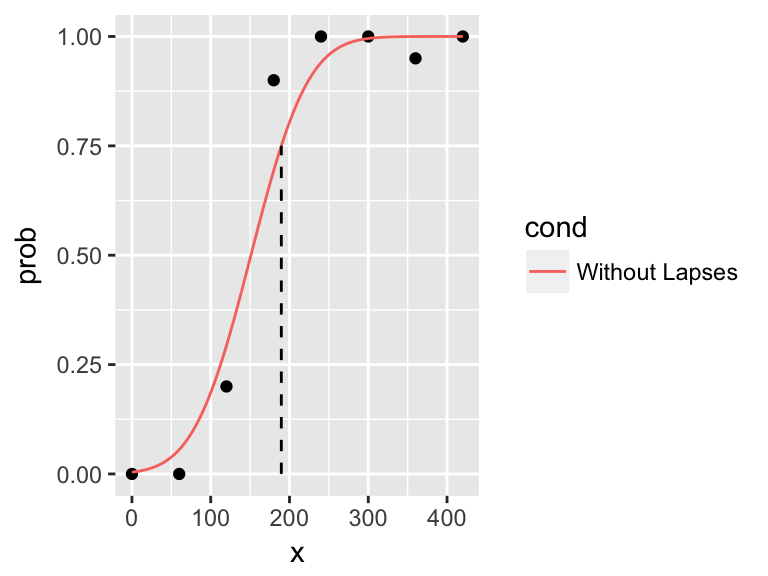As lapses do occur, the fit doesn’t seem good and the threshold biased. The problem is not that the likelihood is not correctly maximized; this weird fit is the one with the maximum likelihood, given the lapses (Wichmann and Hill, 2001). To reassure that the optimization process is not reaching a local maximum, we repeate the fit using differential evolution algoriths (function DEoptim) fitWithoutLapses2 <- quickpsy(dat, x, k, n, prob = .75, optimization = 'DE', parini = list(c(1, 500), c(1, 500), c(0,1)), bootstrap = 'none') curvesWithoutLapses2 <- fitWithoutLapses2$curves %>% mutate(cond = 'Without Lapses')

pWithout2 <- ggplot()+
geom_point(data = fitWithoutLapses2$averages, aes(x = x, y = prob)) + geom_line(data = curvesWithoutLapses, aes(x = x, y = y, color = cond)) + geom_linerange(data = fitWithoutLapses2$thresholds,
aes(x = thre, ymin = 0, ymax = prob), lty =2)
pWithout2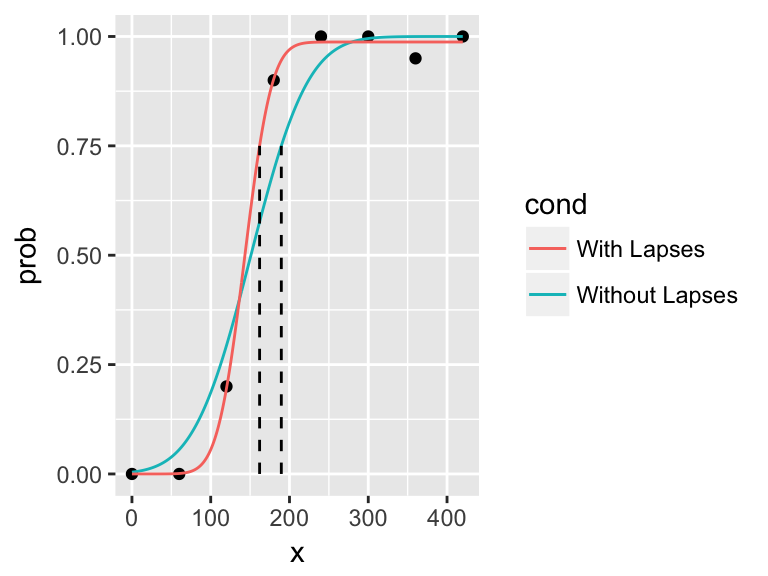and obtain the the same result.

Wichmann and Hill (2001) reported that allowing the upper assymptote to vary — that is, fitting the lapses — eliminates the bias for the threshold, which seems to work in this example

fitWithLapses <- quickpsy(dat, x, k, n, prob = .75, lapses = T)
curvesWithLapses <- fitWithLapses$curves %>% mutate(cond = 'With Lapses') pWithoutWith <- pWithout + geom_line(data = curvesWithLapses, aes(x = x, y = y, color = cond)) + geom_linerange(data = fitWithLapses$thresholds,
aes(x = thre, ymin = 0, ymax = prob), lty =2)
pWithoutWith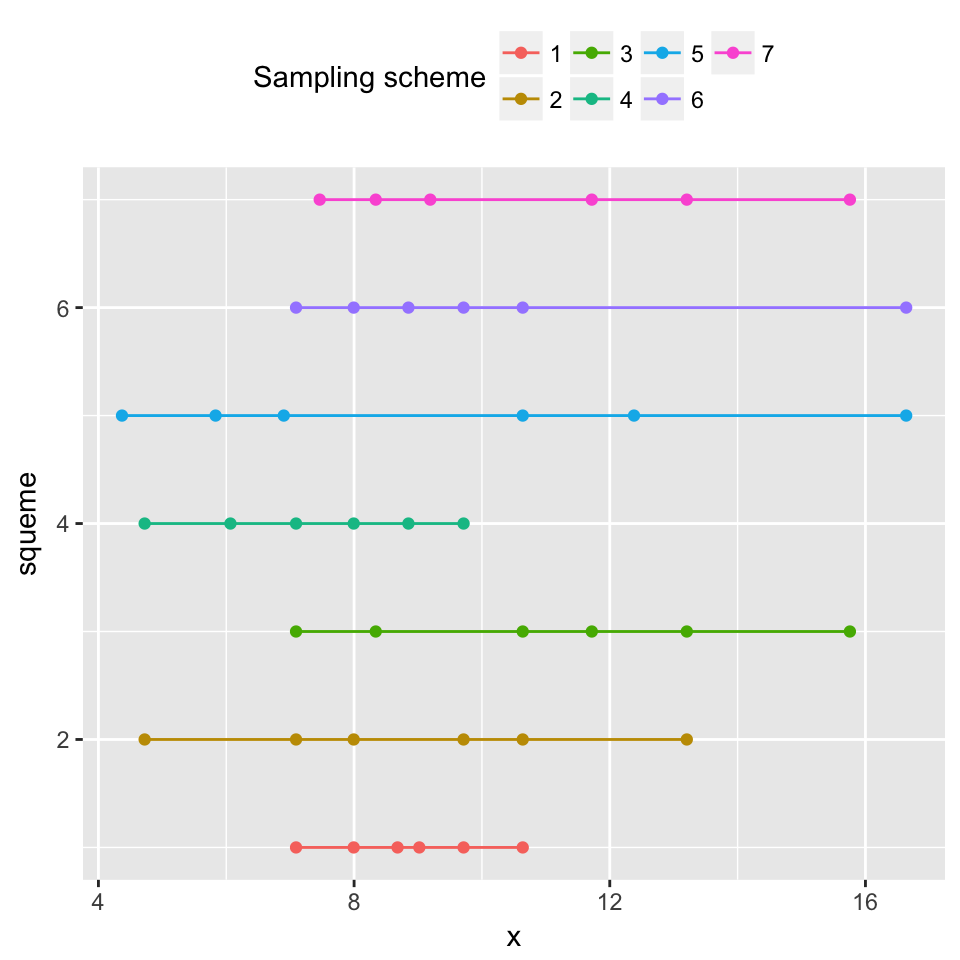## Lapses can bias threshold estimation, a second example

data(qpdat)
fitWithoutLapses <- quickpsy(qpdat, phase, resp,grouping = c(ecc, interval ,participant))

fitWithoutLapses %>% plot()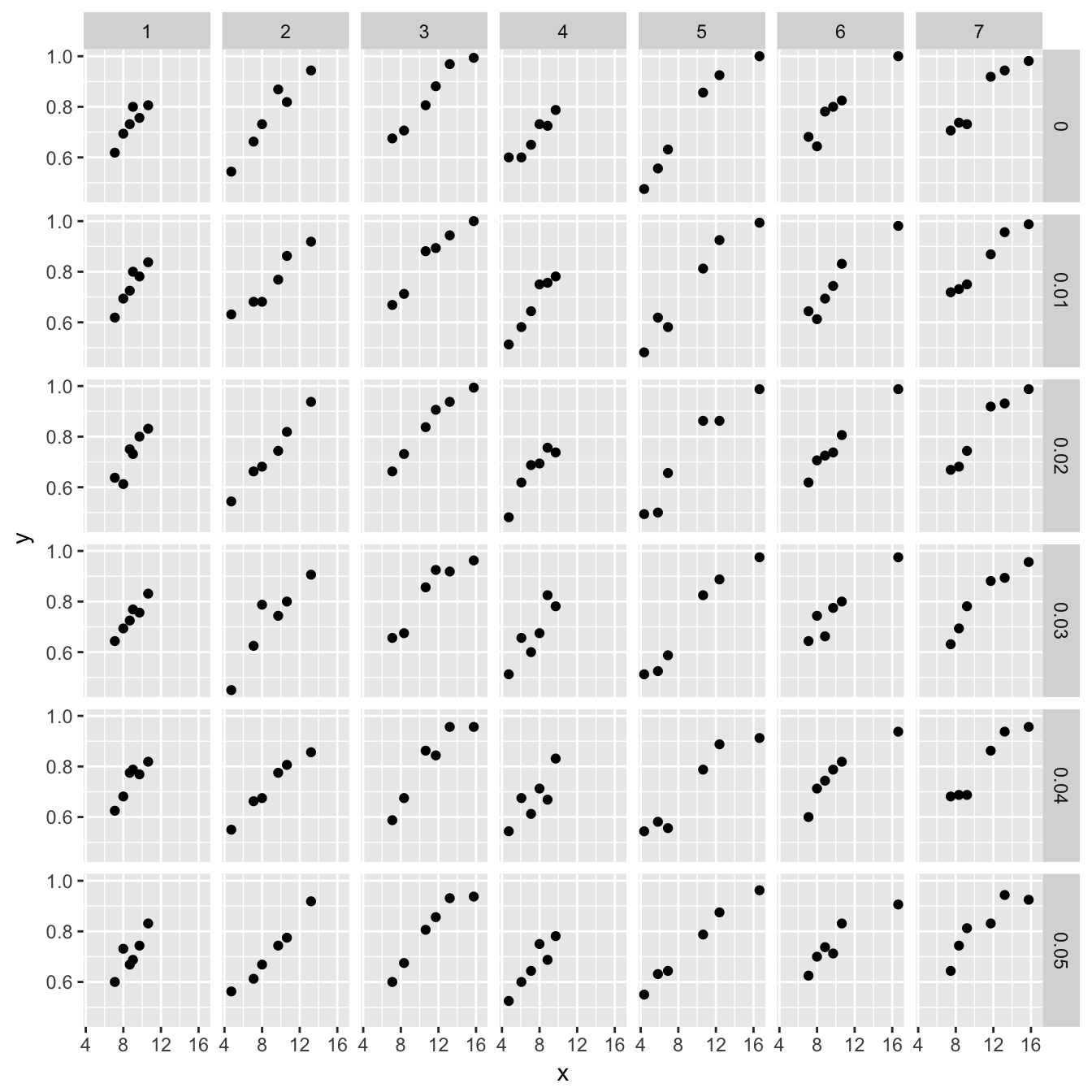Due to lapses, some fits, like the one in the upper left panel for the blue dots, do not seem good, so let’s allow the lapses to vary

fitWithLapses <- quickpsy(qpdat, phase, resp, grouping = .(ecc, interval ,participant), lapses  = T) 
## Error: As y-predictions are not within (0,1), bootstrap should be 'nonparametric'

We obtain an error in the bootstrap. To understand the error we plot the results without bootstraping

fitWithLapses <- quickpsy(qpdat, phase, resp, grouping = c(ecc, interval ,participant), lapses  = T, bootstrap = 'none')

ggplot() +
facet_grid(interval~participant) +
geom_point(data = fitWithLapses$averages, aes(x = phase, y = prob, color = factor(ecc))) + geom_line(data = fitWithLapses$curves,
aes(x = x, y = y, color = factor(ecc))) +
ylim(0, 1.25) # we include ggplot ylim to be able to visualize what is going on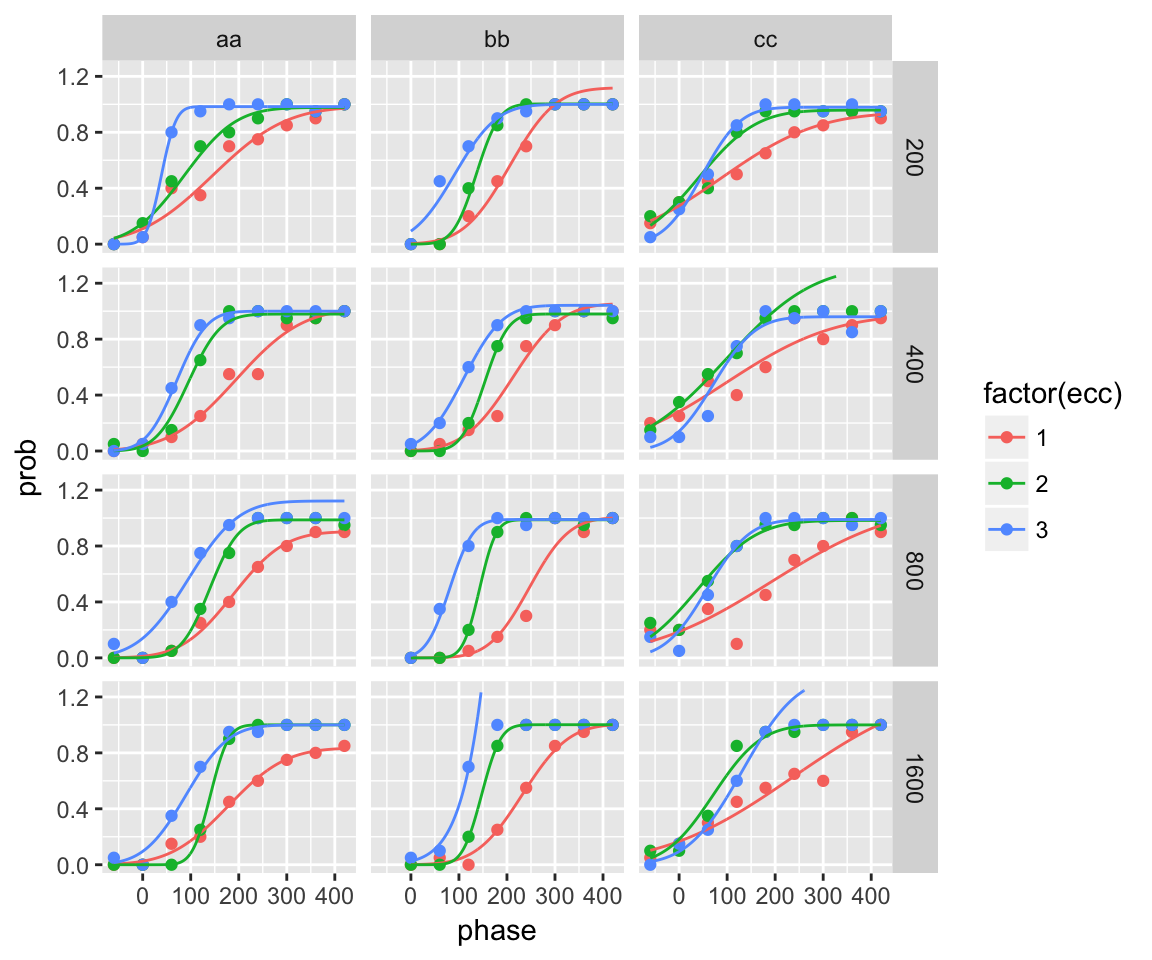The problem is that, as we did not constraint the values of the upper asymptote, the lapse rate is eventually negative and consequently the fitted curves go through probabilities larger than one, which causes that the parametric bootstrap samples cannot be calculated. For this reason, quickpsy indicates that the bootstrap should be non-parametric. We avoid the error by performing a non-parametric bootstrap

fitWithLapses <- quickpsy(qpdat, phase, resp,
grouping = c(ecc, interval ,participant), lapses  = TRUE,
bootstrap = 'nonparametric')

ggplot() +
facet_grid(interval~participant) +
geom_point(data = fitWithLapses$averages, aes(x = phase, y = prob, color = factor(ecc))) + geom_line(data = fitWithLapses$curves,
aes(x = x, y = y, color = factor(ecc))) +
ylim(0, 1.25)Another, possibly better, solution is to perform a parametric bootstrap but constraining the values of the upper asymptote

fitWithLapses <- quickpsy(qpdat, phase, resp,
grouping = c(ecc, interval ,participant), lapses  = T,
parini = list(c(20, 1000), c(20, 1000), c(0, .1)))

fitWithLapses %>% plot()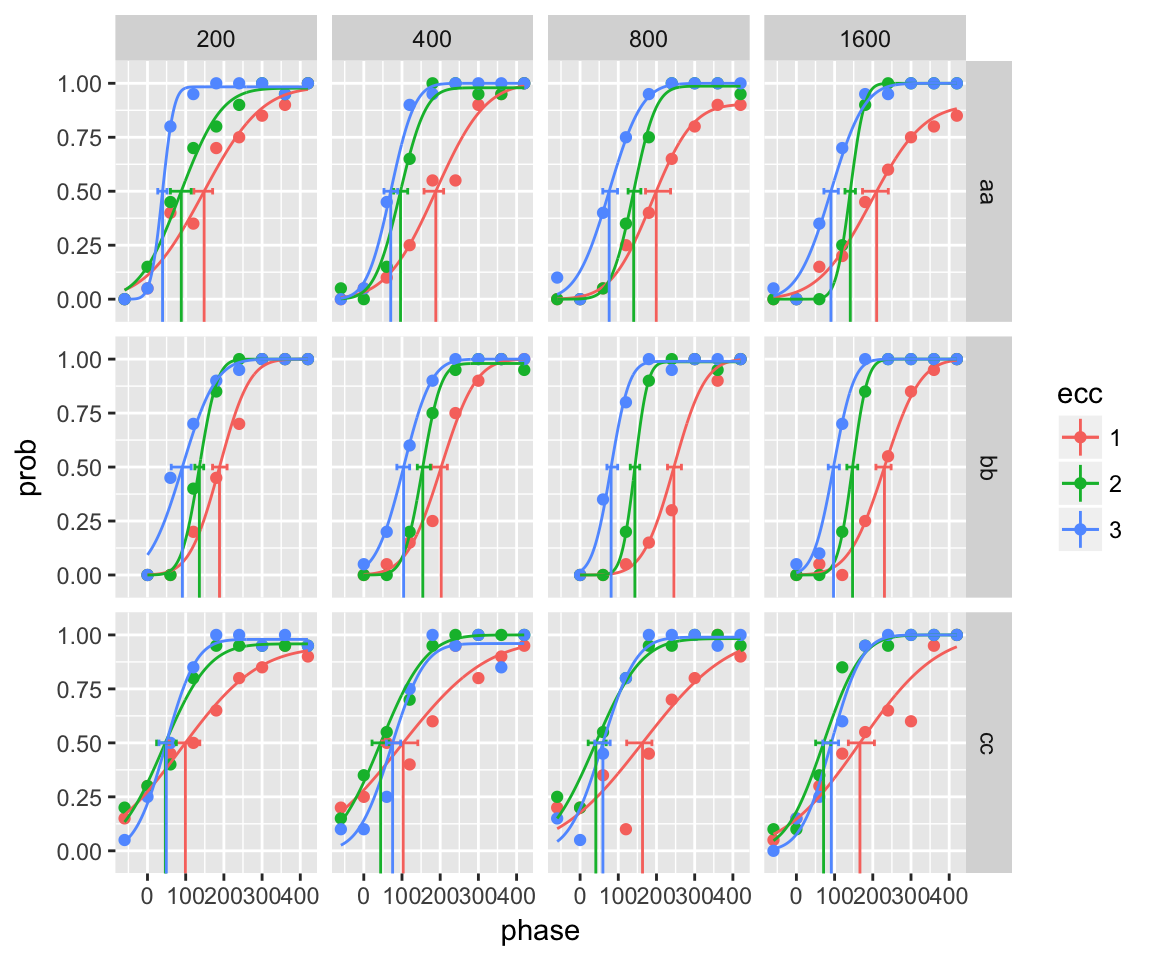## Wichmann and Hill simulations

By using the same simulations as in Wichmann and Hill (2001), here we reproduce the bias of the estimated thresholds due to response lapses (see the original paper for details).

Wichmann and Hill used 7 sampling squemes defined as follows

parweibull <- c(10, 3)
create_xs <- function(i, f) data.frame(squeme = i,  y = f, x = inv_weibull_fun(f, parweibull))
s <- list()
s[] <- create_xs(1, c(.3, .4, .48, .52, .6, .7))
s[] <- create_xs(2, c(.1, .3, .4, .6, .7, .9))
s[] <- create_xs(3, c(.3, .44, .7, .8, .9, .98))
s[] <- create_xs(4, c(.1, .2, .3, .4, .5, .6))
s[] <- create_xs(5, c(.08, .18, .28, .7, .85, .99))
s[] <- create_xs(6, c(.3, .4, .5, .6, .7, .99))
s[] <- create_xs(7, c(.34, .44, .54, .8, .9, .98))
s <- do.call('rbind', s)

ggplot(s, aes(x = x, y = squeme, color = factor(squeme))) +
geom_point() + geom_line() +
labs(color = 'Sampling scheme') + theme(legend.position = 'top')They generated parametric bootstrap samples of data coming from a psychometric function with the shape of a weibull function with parameters $$10$$ and $$3$$, $$guess=0.5$$ and variable $$\lambda$$ (directly related to the lapses).

For each value of the independent variable $$x$$, we simulated 160 trials (in the original paper 20, 40 and 80 trials were also used).

create_sim_dat <- function(d) {
psychometric_fun <- create_psy_fun(weibull_fun, .5, d$lambda) ypred <- psychometric_fun(d$x, parweibull)
k <- rbinom(length(d$x), d$n, ypred)
data.frame(x = d$x, k = k, n = d$n , y = k/d$n) } simdat <- merge(s, expand.grid(n = 160, sample = 1:10, lambda = seq(0,.05, .01))) %>% group_by(squeme, n, sample, lambda) %>% do(create_sim_dat(.)) # we use only 10 samples to increase decrease the computation time To illustrate the simulated data, we plot the first sample for each sampling scheme (columns) and each value of $$\lambda$$ (rows) p <- ggplot(simdat %>% filter(sample == 1)) + facet_grid(lambda ~ squeme) + geom_point(aes(x = x, y = y)) p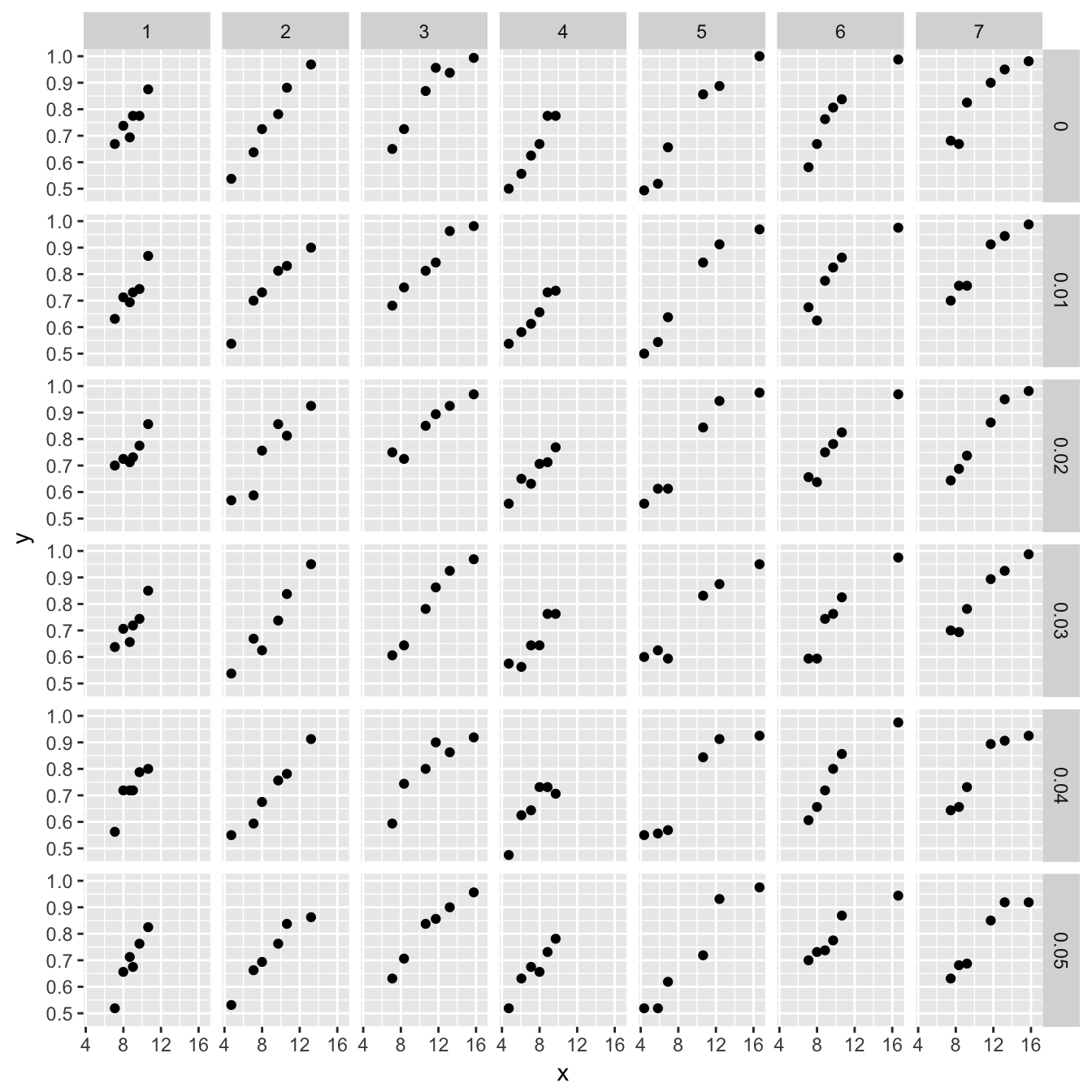We use quickpsy to fit a weibull function to each condition using $$\lambda = 0$$ fit <- quickpsy(simdat, x, k, n, within = .(squeme, lambda, sample), fun = weibull_fun, bootstrap = 'none', guess =.5, lapses = 0) # we use 'none' for the bootstap because we are doing an explicit bootstrap # (we are fitting by sample), that is, we don't need to bootstrap each of our samples and for the sake of illustration show the fitted psychometric function for the first sample p + geom_line(data = fit$curves %>% filter(sample == 1),
aes(x = x, y =y))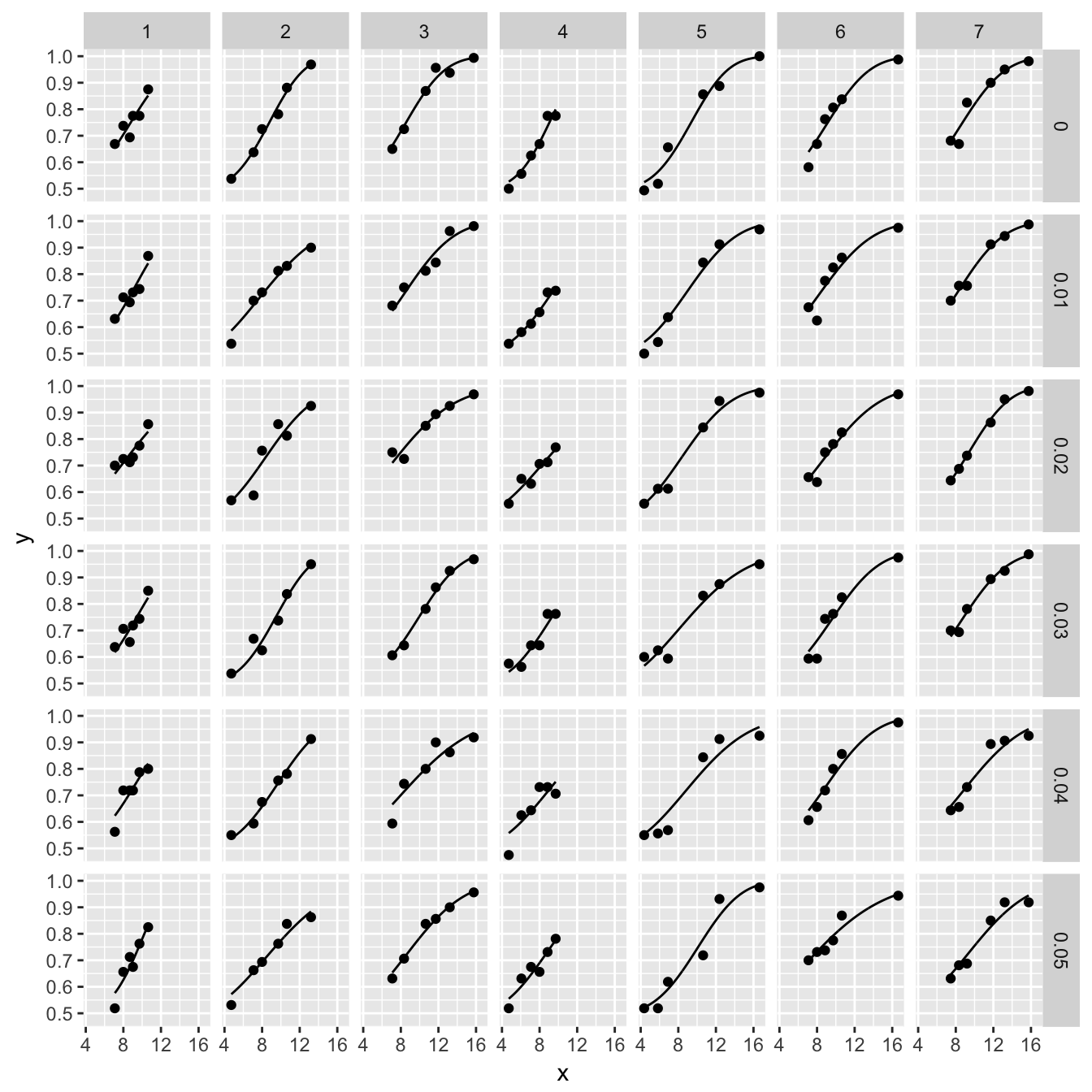# we use the function curves instead of fit$curves because we want to # define the range of the curves manually Replicating Wichmann and Hill, we show that the thresholds are overstimated as $$\lambda$$ increases (they showed that the slope is also biased) thre <- fit$thresholds %>% group_by(squeme, lambda) %>%
summarise (threshold = mean(thre))

real_threshold <- inv_weibull_fun((.75 - .5) / (1 - .5 - 0), parweibull)

ggplot(thre) +
geom_point(aes(x = lambda, y = threshold, color = factor(squeme)), size = 4) +
geom_hline(yintercept = real_threshold, lty = 2)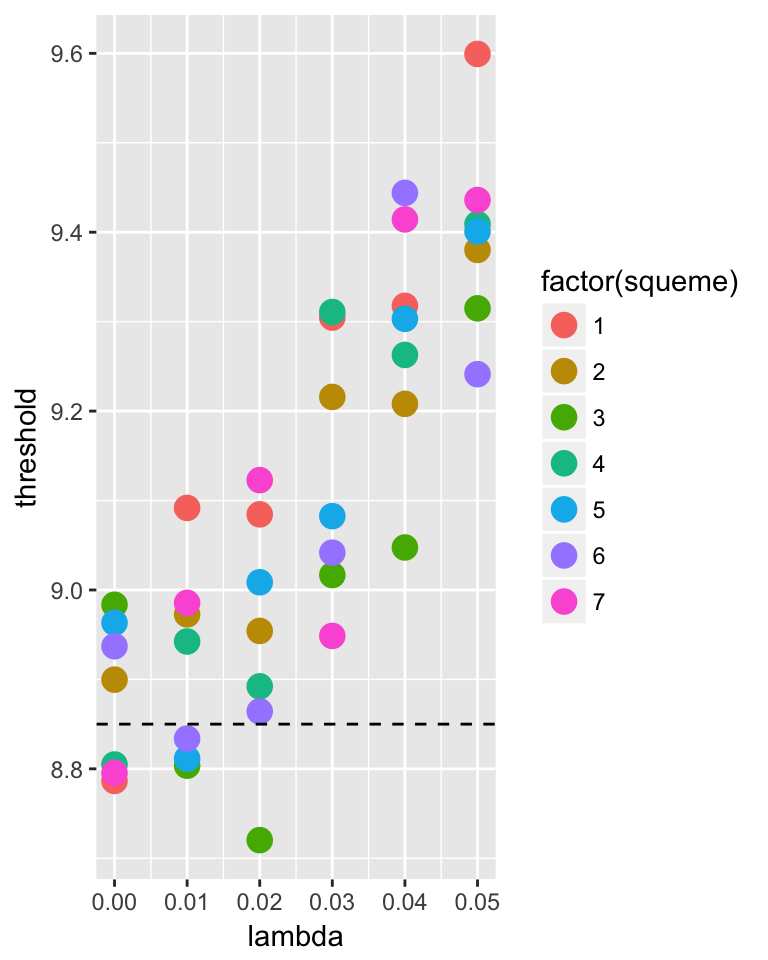Wichmann and Hill (2001) reported that allowing $$\lambda$$ to vary within a given small window eliminates the bias for the threshold, but consistently with Prins (2012) we were not able to replicate this finding

fit_lapses <- quickpsy(simdat, x, k, n, within = .(squeme, lambda, sample),
fun = weibull_fun, bootstrap = 'none', guess =.5, lapses = T,
parini = list(c(1,30), c(1,10), c(0,.05))) # we introduce the min and max value for each parameter

thre_lapses <- fit_lapses\$thresholds %>% group_by(squeme, lambda) %>%
summarise (threshold = mean(thre))

ggplot(thre_lapses) +
geom_point(aes(x = lambda, y = threshold, color = factor(squeme)), size = 4) +
geom_hline(yintercept = real_threshold, lty = 2)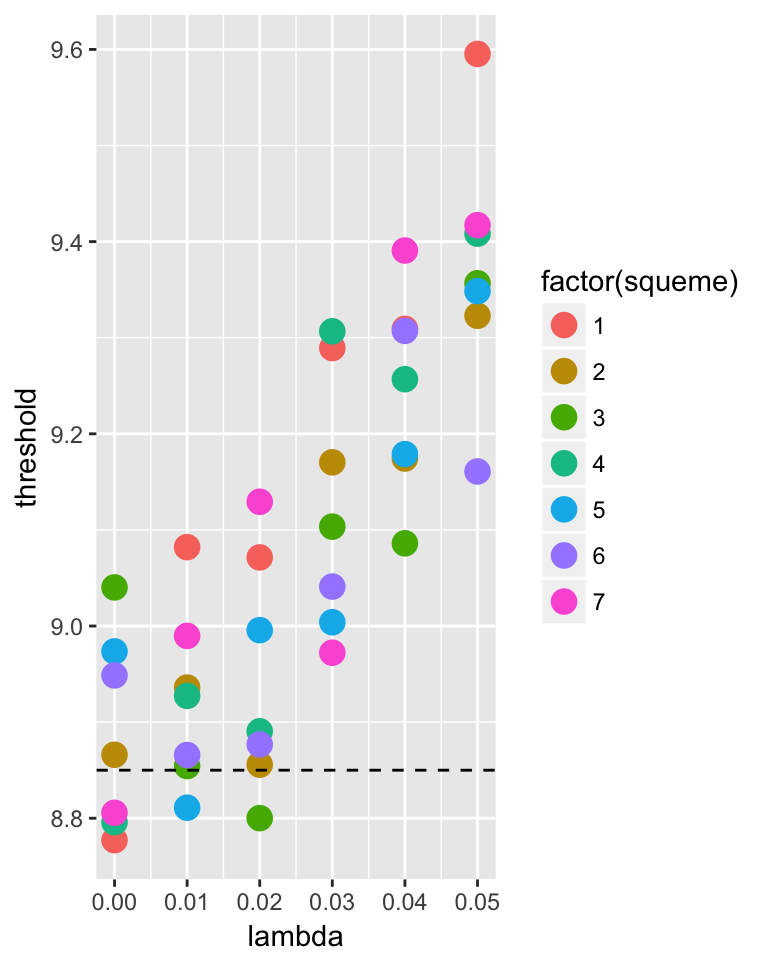So, fitting the lapses does not always eliminate the bias in threshold estimation.